# Tag: simplesIn his paper Cyclotomy and analytic geometry over $\mathbb{F}_1$ Yuri I. Manin sketches and compares four approaches to the definition of a geometry over $\mathbb{F}_1$, the elusive field with one element.

He writes : “Preparing a colloquium talk in Paris, I have succumbed to the temptation to associate them with some dominant trends in the history of art.”

Remember that the search for the absolute point $\pmb{spec}(\mathbb{F}_1)$ originates from the observation that $\pmb{spec}(\mathbb{Z})$, the set of all prime numbers together with $0$, is too large to serve as the terminal object in Grothendieck’s theory of commutative schemes. The last couple of years have seen a booming industry of proposals, to the extent that Javier Lopez Pena and Oliver Lorscheid decided they had to draw a map of $\mathbb{F}_1$-land.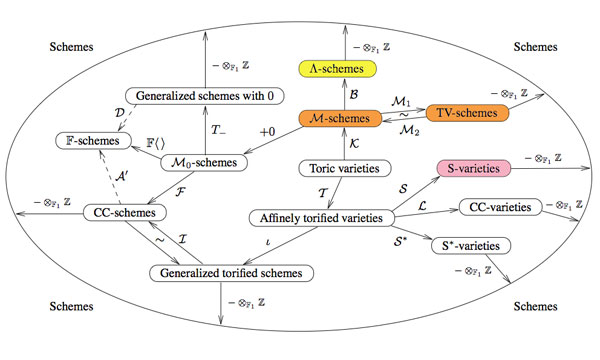Manin only discusses the colored proposals (TV=Toen-Vaquie, M=Deitmar, S=Soule and $\Lambda$=Borger) and compares them to these art-history trends.

Toen and Vaquie : Abstract ExpressionismIn Under $\pmb{spec}(\mathbb{Z})$ Bertrand Toen and Michel Vaquie argue that geometry over $\mathbb{F}_1$ is a special case of algebraic geometry over a symmetric monoidal category, taking the simplest example namely sets and direct products. Probably because of its richness and abstract nature, Manin associates this approach to Abstract Expressionism (a.o. Karel Appel, Jackson Pollock, Mark Rothko, Willem de Kooning).

Deitmar : Minimalism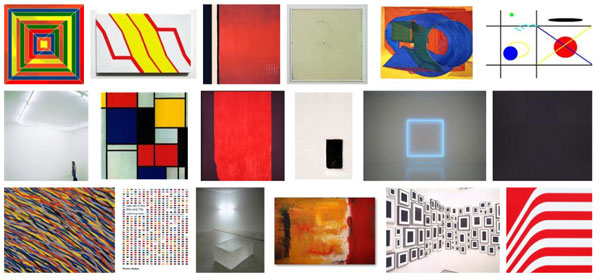Because monoids are the ‘commutative algebras’ in sets with direct products, an equivalent proposal is that of Anton Deitmar in Schemes over $\mathbb{F}_1$ in which the basic affine building blocks are spectra of monoids, topological spaces whose points are submonoids satisfying a primeness property. Because Deitmar himself calls this approach a ‘minimalistic’ one it is only natural to associate to it Minimalism where the work is stripped down to its most fundamental features. Prominent artists associated with this movement include Donald Judd, John McLaughlin, Agnes Martin, Dan Flavin, Robert Morris, Anne Truitt, and Frank Stella.

Soule : Critical Realismin Les varietes sur le corps a un element Christophe Soule defines varieties over $\mathbb{F}_1$ to be specific schemes $X$ over $\mathbb{Z}$ together with a form of ‘descent data’ as well as an additional $\mathbb{C}$-algebra, morally the algebra of functions on the real place. Because of this Manin associates to it Critical Realism in philosophy. There are also ‘realism’ movements in art such as American Realism (o.a. Edward Hopper and John Sloan).

Borger : FuturismJames Borger’s paper Lambda-rings and the field with one element offers a totally new conception of the descent data from $\mathbb{Z}$ to $\mathbb{F}_1$, namely that of a $\lambda$-ring in the sense of Grothendieck. Because Manin expects this approach to lead to progress in the field, he connects it to Futurism, an artistic and social movement that originated in Italy in the early 20th century.

Here’s a tiny problem illustrating our limited knowledge of finite fields : “Imagine an infinite queue of Knights ${ K_1,K_2,K_3,\ldots }$, waiting to be seated at the unit-circular table. The master of ceremony (that is, you) must give Knights $K_a$ and $K_b$ a place at an odd root of unity, say $\omega_a$ and $\omega_b$, such that the seat at the odd root of unity $\omega_a \times \omega_b$ must be given to the Knight $K_{a \otimes b}$, where $a \otimes b$ is the Nim-multiplication of $a$ and $b$. Which place would you offer to Knight $K_{16}$, or Knight $K_n$, or, if you’re into ordinals, Knight $K_{\omega}$?”

What does this have to do with finite fields? Well, consider the simplest of all finite field $\mathbb{F}_2 = { 0,1 }$ and consider its algebraic closure $\overline{\mathbb{F}_2}$. Last year, we’ve run a series starting here, identifying the field $\overline{\mathbb{F}_2}$, following John H. Conway in ONAG, with the set of all ordinals smaller than $\omega^{\omega^{\omega}}$, given the Nim addition and multiplication. I know that ordinal numbers may be intimidating at first, so let’s just restrict to ordinary natural numbers for now. The Nim-addition of two numbers $n \oplus m$ can be calculated by writing the numbers n and m in binary form and add them without carrying. For example, $9 \oplus 1 = 1001+1 = 1000 = 8$. Nim-multiplication is slightly more complicated and is best expressed using the so-called Fermat-powers $F_n = 2^{2^n}$. We then demand that $F_n \otimes m = F_n \times m$ whenever $m < F_n$ and $F_n \otimes F_n = \frac{3}{2}F_n$. Distributivity wrt. $\oplus$ can then be used to calculate arbitrary Nim-products. For example, $8 \otimes 3 = (4 \otimes 2) \otimes (2 \oplus 1) = (4 \otimes 3) \oplus (4 \otimes 2) = 12 \oplus 8 = 4$. Conway’s remarkable result asserts that the ordinal numbers, equipped with Nim addition and multiplication, form an algebraically closed field of characteristic two. The closure $\overline{\mathbb{F}_2}$ is identified with the subfield of all ordinals smaller than $\omega^{\omega^{\omega}}$. For those of you who don’t feel like going transfinite, the subfield $~(\mathbb{N},\oplus,\otimes)$ is identified with the quadratic closure of $\mathbb{F}_2$.

The connection between $\overline{\mathbb{F}_2}$ and the odd roots of unity has been advocated by Alain Connes in his talk before a general public at the IHES : “L’ange de la géométrie, le diable de l’algèbre et le corps à un élément” (the angel of geometry, the devil of algebra and the field with one element). He describes its content briefly in this YouTube-video

At first it was unclear to me which ‘coupling-problem’ Alain meant, but this has been clarified in his paper together with Caterina Consani Characteristic one, entropy and the absolute point. The non-zero elements of $\overline{\mathbb{F}_2}$ can be identified with the set of all odd roots of unity. For, if x is such a unit, it belongs to a finite subfield of the form $\mathbb{F}_{2^n}$ for some n, and, as the group of units of any finite field is cyclic, x is an element of order $2^n-1$. Hence, $\mathbb{F}_{2^n}- { 0 }$ can be identified with the set of $2^n-1$-roots of unity, with $e^{2 \pi i/n}$ corresponding to a generator of the unit-group. So, all elements of $\overline{\mathbb{F}_2}$ correspond to an odd root of unity. The observation that we get indeed all odd roots of unity may take you a couple of seconds (( If m is odd, then (2,m)=1 and so 2 is a unit in the finite cyclic group $~(\mathbb{Z}/m\mathbb{Z})^*$ whence $2^n = 1 (mod~m)$, so the m-roots of unity lie within those of order $2^n-1$ )).

Assuming we succeed in fixing a one-to-one correspondence between the non-zero elements of $\overline{\mathbb{F}_2}$ and the odd roots of unity $\mu_{odd}$ respecting multiplication, how can we recover the addition on $\overline{\mathbb{F}_2}$? Well, here’s Alain’s coupling function, he ties up an element x of the algebraic closure to the element s(x)=x+1 (and as we are in characteristic two, this is an involution, so also the element tied up to x+1 is s(x+1)=(x+1)+1=x. The clue being that multiplication together with the coupling map s allows us to compute any sum of two elements as $x+y=x \times s(\frac{y}{x}) = x \times (\frac{y}{x}+1)$.
For example, all information about the finite field $\mathbb{F}_{2^4}$ is encoded in this identification with the 15-th roots of unity, together with the pairing s depicted as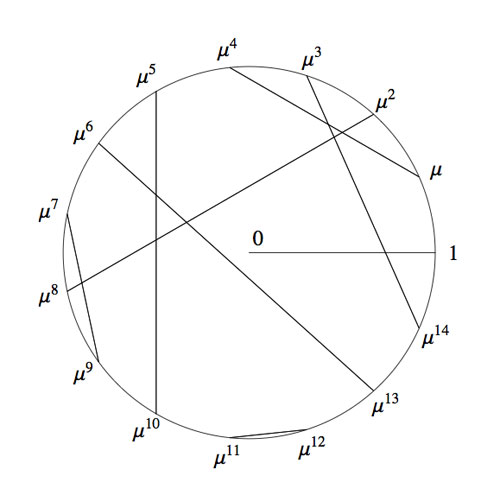Okay, we now have two identifications of the algebraic closure $\overline{\mathbb{F}_2}$ : the smaller ordinals equipped with Nim addition and Nim multiplication and the odd roots of unity with complex-multiplication and the Connes-coupling s. The question we started from asks for a general recipe to identify these two approaches.

To those of you who are convinced that finite fields (LOL, even characteristic two!) are objects far too trivial to bother thinking about : as far as I know, NOBODY knows how to do this explicitly, even restricting the ordinals to merely the natural numbers!

Please feel challenged! To get you started, I’ll show you how to place the first 15 Knights and give you a procedure (though far from explicit) to continue. Here’s the Nim-picture compatible with that above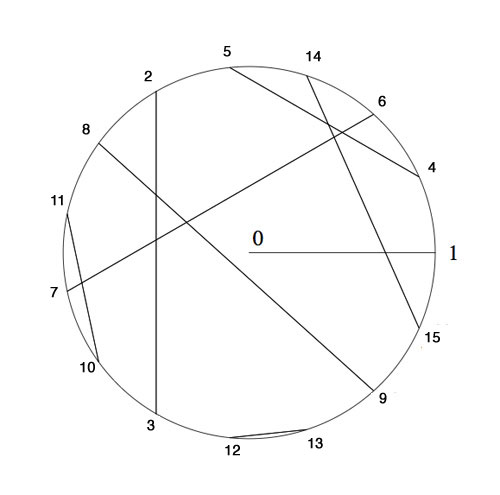To verify this, and to illustrate the general strategy, I’d better hand you the Nim-tables of the first 16 numbers. Here they are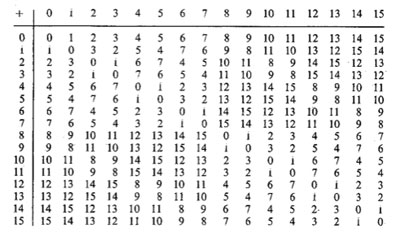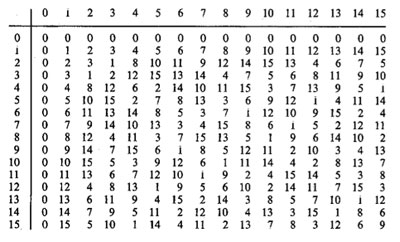It is known that the finite subfields of $~(\mathbb{N},\oplus,\otimes)$ are precisely the sets of numbers smaller than the Fermat-powers $F_n$. So, the first one is all numbers smaller than $F_1=4$ (check!). The smallest generator of the multiplicative group (of order 3) is 2, so we take this to correspond to the unit-root $e^{2 \pi i/3}$. The next subfield are all numbers smaller than $F_2 = 16$ and its multiplicative group has order 15. Now, choose the smallest integer k which generates this group, compatible with the condition that $k^{\otimes 5}=2$. Verify that this number is 4 and that this forces the identification and coupling given above.

The next finite subfield would consist of all natural numbers smaller than $F_3=256$. Hence, in this field we are looking for the smallest number k generating the multiplicative group of order 255 satisfying the extra condition that $k^{\otimes 17}=4$ which would fix an identification at that level. Then, the next level would be all numbers smaller than $F_4=65536$ and again we would like to find the smallest number generating the multiplicative group and such that the appropriate power is equal to the aforementioned k, etc. etc.

Can you give explicit (even inductive) formulae to achieve this? I guess even the problem of placing Knight 16 will give you a couple of hours to think about… (to be continued).

I really like Matilde Marcolli’s idea to use some of Jackson Pollock’s paintings as metaphors for noncommutative spaces. In her talk she used this painting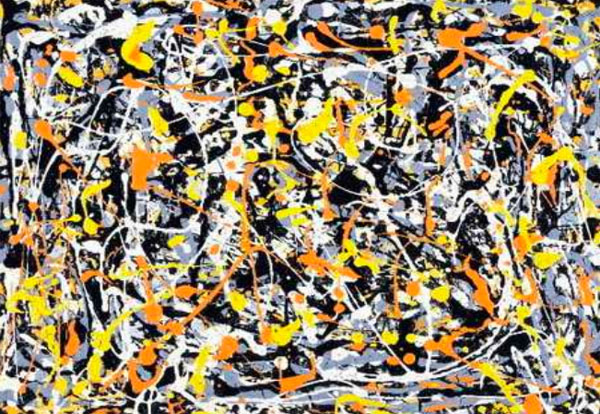and refered to it (as did I in my post) as : Jackson Pollock “Untitled N.3”. Before someone writes a post ‘The Pollock noncommutative space hoax’ (similar to my own post) let me point out that I am well aware of the controversy surrounding this painting.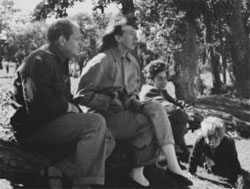This painting is among 32 works recently discovered and initially attributed to Pollock.
In fact, I’ve already told part of the story in Doodles worth millions (or not)? (thanks to PD1). The story involves the people on the right : from left to right, Jackson Pollock, his wife Lee Krasner, Mercedes Matter and her son Alex Matter.

Alex Matter, whose father, Herbert, and mother, Mercedes, were artists and friends of Jackson Pollock, discovered after his mother died a group of small drip paintings in a storage locker in Wainscott, N.Y. which he believed to be authentic Pollocks.

Read the post mentioned above if you want to know how mathematics screwed up his plan, or much better, reed the article Anatomy of the Jackson Pollock controversy by Stephen Litt.

So, perhaps the painting above was not the smartest choice, but we could take any other genuine Pollock ‘drip-painting’, a technique he taught himself towards the end of 1946 to make an image by splashing, pouring, sloshing colors onto the canvas. Typically, such a painting consists of blops of paint, connected via thin drip-lines.

What does this have to do with noncommutative geometry? Well, consider the blops as ‘points’. In commutative geometry, distinct points cannot share tangent information ((technically : a commutative semi-local ring splits as the direct sum of local rings and this does no longer hold for a noncommutative semi-local ring)). In the noncommutative world though, they can!, or if you want to phrase it like this, noncommutative points ‘can talk to each other’. And, that’s what we cherish in those drip-lines.

But then, if two points share common tangent informations, they must be awfully close to each other… so one might imagine these Pollock-lines to be strings holding these points together. Hence, it would make more sense to consider the ‘Pollock-quotient-painting’, that is, the space one gets after dividing out the relation ‘connected by drip-lines’ ((my guess is that Matilde thinks of the lines as the action of a group on the points giving a topological horrible quotient space, and thats precisely where noncommutative geometry shines)).

For this reason, my own mental picture of a genuinely noncommutative space ((that is, the variety corresponding to a huge noncommutative algebra such as free algebras, group algebras of arithmetic groups or fundamental groups)) looks more like the picture below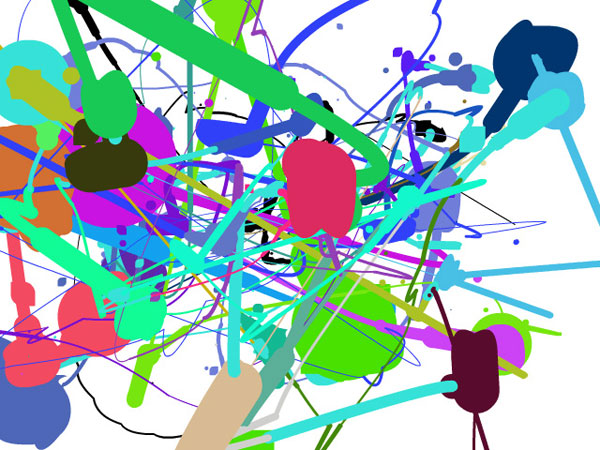The colored blops you see are really sets of points which you might view as, say, a FacebookGroup ((technically, think of them as the connected components of isomorphism classes of finite dimensional simple representations of your favorite noncommutative algebra)). Some chatter may occur between two distinct FacebookGroups, the more chatter the thicker the connection depicted ((technically, the size of the connection is the dimension of the ext-group between generic simples in the components)). Now, there are some tiny isolated spots (say blue ones in the upper right-hand quadrant). These should really be looked at as remote clusters of noncommutative points (sharing no (tangent) information whatsoever with the blops in the foregound). If we would zoom into them beyond the Planck scale (if I’m allowed to say a bollock-word in a Pollock-post) they might reveal again a whole universe similar to the interconnected blops upfront.

The picture was produced using the fabulous Pollock engine. Just use your mouse to draw and click to change colors in order to produce your very own noncommutative space!

For the mathematicians still around, this may sound like a lot of Pollock-bollocks but can be made precise. See my note Noncommutative geometry and dual coalgebras for a very terse reading. Now that coalgebras are gaining popularity, I really should write a more readable account of it, including some fanshi-wanshi examples…Last time we did recall Cantor’s addition and multiplication on ordinal numbers. Note that we can identify an ordinal number $\alpha$ with (the order type of) the set of all strictly smaller ordinals, that is, $\alpha = { \alpha’~:~\alpha’ < \alpha }$. Given two ordinals $\alpha$ and $\beta$ we will denote their Cantor-sums and products as $[ \alpha + \beta]$ and $[\alpha . \beta]$.

The reason for these square brackets is that John Conway constructed a well behaved nim-addition and nim-multiplication on all ordinals $\mathbf{On}_2$ by imposing the ‘simplest’ rules which make $\mathbf{On}_2$ into a field. By this we mean that, in order to define the addition $\alpha + \beta$ we must have constructed before all sums $\alpha’ + \beta$ and $\alpha + \beta’$ with $\alpha’ < \alpha$ and $\beta’ < \beta$. If + is going to be a well-defined addition on $\mathbf{On}_2$ clearly $\alpha + \beta$ cannot be equal to one of these previously constructed sums and the ‘simplicity rule’ asserts that we should take $\alpha+\beta$ the least ordinal different from all these sums $\alpha’+\beta$ and $\alpha+\beta’$. In symbols, we define

$\alpha+ \beta = \mathbf{mex} { \alpha’+\beta,\alpha+ \beta’~|~\alpha’ < \alpha, \beta’ < \beta }$

where $\mathbf{mex}$ stands for ‘minimal excluded value’. If you’d ever played the game of Nim you will recognize this as the Nim-addition, at least when $\alpha$ and $\beta$ are finite ordinals (that is, natural numbers) (to nim-add two numbers n and m write them out in binary digits and add without carrying). Alternatively, the nim-sum n+m can be found applying the following two rules :

• the nim-sum of a number of distinct 2-powers is their ordinary sum (e.g. $8+4+1=13$, and,
• the nim-sum of two equal numbers is 0.

So, all we have to do is to write numbers n and m as sums of two powers, scratch equal terms and add normally. For example, $13+7=(8+4+1)+(4+2+1)=8+2=10$ (of course this is just digital sum without carry in disguise).Here’s the beginning of the nim-addition table on ordinals. For example, to define $13+7$ we have to look at all values in the first 7 entries of the row of 13 (that is, ${ 13,12,15,14,9,8,11 }$) and the first 13 entries in the column of 7 (that is, ${ 7,6,5,4,3,2,1,0,15,14,13,12,11 }$) and find the first number not included in these two sets (which is indeed $10$).

In fact, the above two rules allow us to compute the nim-sum of any two ordinals. Recall from last time that every ordinal can be written uniquely as as a finite sum of (ordinal) 2-powers :
$\alpha = [2^{\alpha_0} + 2^{\alpha_1} + \ldots + 2^{\alpha_k}]$, so to determine the nim-sum $\alpha+\beta$ we write both ordinals as sums of ordinal 2-powers, delete powers appearing twice and take the Cantor ordinal sum of the remaining sum.Nim-multiplication of ordinals is a bit more complicated. Here’s the definition as a minimal excluded value

$\alpha.\beta = \mathbf{mex} { \alpha’.\beta + \alpha.\beta’ – \alpha’.\beta’ }$

for all $\alpha’ < \alpha, \beta’ < \beta$. The rationale behind this being that both $\alpha-\alpha’$ and $\beta – \beta’$ are non-zero elements, so if $\mathbf{On}_2$ is going to be a field under nim-multiplication, their product should be non-zero (and hence strictly greater than 0), that is, $~(\alpha-\alpha’).(\beta-\beta’) > 0$. Rewriting this we get $\alpha.\beta > \alpha’.\beta+\alpha.\beta’-\alpha’.\beta’$ and again the ‘simplicity rule’ asserts that $\alpha.\beta$ should be the least ordinal satisfying all these inequalities, leading to the $\mathbf{mex}$-definition above. The table gives the beginning of the nim-multiplication table for ordinals. For finite ordinals n and m there is a simple 2 line procedure to compute their nim-product, similar to the addition-rules mentioned before :

• the nim-product of a number of distinct Fermat 2-powers (that is, numbers of the form $2^{2^n}$) is their ordinary product (for example, $16.4.2=128$), and,
• the square of a Fermat 2-power is its sesquimultiple (that is, the number obtained by multiplying with $1\frac{1}{2}$ in the ordinary sense). That is, $2^2=3,4^2=6,16^2=24,…$

Using these rules, associativity and distributivity and our addition rules it is now easy to work out the nim-multiplication $n.m$ : write out n and m as sums of (multiplications by 2-powers) of Fermat 2-powers and apply the rules. Here’s an example

$5.9=(4+1).(4.2+1)=4^2.2+4.2+4+1=6.2+8+4+1=(4+2).2+13=4.2+2^2+13=8+3+13=6$

Clearly, we’d love to have a similar procedure to calculate the nim-product $\alpha.\beta$ of arbitrary ordinals, or at least those smaller than $\omega^{\omega^{\omega}}$ (recall that Conway proved that this ordinal is isomorphic to the algebraic closure $\overline{\mathbb{F}}_2$ of the field of two elements). From now on we restrict to such ‘small’ ordinals and we introduce the following special elements :

$\kappa_{2^n} = [2^{2^{n-1}}]$ (these are the Fermat 2-powers) and for all primes $p > 2$ we define
$\kappa_{p^n} = [\omega^{\omega^{k-1}.p^{n-1}}]$ where $k$ is the number of primes strictly smaller than $p$ (that is, for p=3 we have k=1, for p=5, k=2 etc.).

Again by associativity and distributivity we will be able to multiply two ordinals $< \omega^{\omega^{\omega}}$ if we know how to multiply a product

$[\omega^{\alpha}.2^{n_0}].[\omega^{\beta}.2^{m_0}]$ with $\alpha,\beta < [\omega^{\omega}]$ and $n_0,m_0 \in \mathbb{N}$.

Now, $\alpha$ can be written uniquely as $[\omega^t.n_t+\omega^{t-1}.n_{t-1}+\ldots+\omega.n_2 + n_1]$ with t and all $n_i$ natural numbers. Write each $n_k$ in base $p$ where $p$ is the $k+1$-th prime number, that is, we have for $n_0,n_1,\ldots,n_t$ an expression

$n_k=[\sum_j p^j.m(j,k)]$ with $0 \leq m(j,k) < p$

The point of all this is that any of the special elements we want to multiply can be written as a unique expression as a decreasing product

$[\omega^{\alpha}.2^{n_0}] = [ \prod_q \kappa_q^m(q) ]$

where $q$ runs over all prime powers. The crucial fact now is that for this decreasing product we have a rule similar to addition of 2-powers, that is Conway-products coincide with the Cantor-products

$[ \prod_q \kappa_q^m(q) ] = \prod_q \kappa_q^m(q)$

But then, using associativity and commutativity of the Conway-product we can ‘nearly’ describe all products $[\omega^{\alpha}.2^{n_0}].[\omega^{\beta}.2^{m_0}]$. The remaining problem being that it may happen that for some q we will end up with an exponent $m(q)+m(q’)>p$. But this can be solved if we know how to take p-powers. The rules for this are as follows

$~(\kappa_{2^n})^2 = \kappa_{2^n} + \prod_{1 \leq i < n} \kappa_{2^i}$, for 2-powers, and,

$~(\kappa_{p^n})^p = \kappa_{p^{n-1}}$ for a prime $p > 2$ and for $n \geq 2$, and finally

$~(\kappa_p)^p = \alpha_p$ for a prime $p > 2$, where $\alpha_p$ is the smallest ordinal $< \kappa_p$ which cannot be written as a p-power $\beta^p$ with $\beta < \kappa_p$. Summarizing : if we will be able to find these mysterious elements $\alpha_p$ for all prime numbers p, we are able to multiply in $[\omega^{\omega^{\omega}}]=\overline{\mathbb{F}}_2$.

Let us determine the first one. We have that $\kappa_3 = \omega$ so we are looking for the smallest natural number $n < \omega$ which cannot be written in num-multiplication as $n=m^3$ for $m < \omega$ (that is, also $m$ a natural number). Clearly $1=1^3$ but what about 2? Can 2 be a third root of a natural number wrt. nim-multiplication? From the tabel above we see that 2 has order 3 whence its cube root must be an element of order 9. Now, the only finite ordinals that are subfields of $\mathbf{On}_2$ are precisely the Fermat 2-powers, so if there is a finite cube root of 2, it must be contained in one of the finite fields $[2^{2^n}]$ (of which the mutiplicative group has order $2^{2^n}-1$ and one easily shows that 9 cannot be a divisor of any of the numbers $2^{2^n}-1$, that is, 2 doesn’t have a finte 3-th root in nim! Phrased differently, we found our first mystery number $\alpha_3 = 2$. That is, we have the marvelous identity in nim-arithmetic

$\omega^3 = 2$

Okay, so what is $\alpha_5$? Well, we have $\kappa_5 = [\omega^{\omega}]$ and we have to look for the smallest ordinal which cannot be written as a 5-th root. By inspection of the finite nim-table we see that 1,2 and 3 have 5-th roots in $\omega$ but 4 does not! The reason being that 4 has order 15 (check in the finite field ) and 25 cannot divide any number of the form $2^{2^n}-1$. That is, $\alpha_5=4$ giving another crazy nim-identity

$~(\omega^{\omega})^5 = 4$

And, surprises continue to pop up… Conway showed that $\alpha_7 = \omega+1$ giving the nim-identity $~(\omega^{\omega^2})^7 = \omega+1$. The proof of this already uses some clever finite field arguments. Because 7 doesn’t divide any number $2^{2^n}-1$, none of the finite subfields $[2^{2^n}]$ contains a 7-th root of unity, so the 7-power map is injective whence surjective, so all finite ordinal have finite 7-th roots! That is, $\alpha_7 \geq \omega$. Because $\omega$ lies in a cubic extension of the finite field , the field generated by $\omega$ has 64 elements and so its multiplicative group is cyclic of order 63 and as $\omega$ has order 9, it must be a 7-th power in this field. But, as the only 7th powers in that field are precisely the powers of $\omega$ and by inspection $\omega+1$ is not a 7-th power in that field (and hence also not in any field extension obtained by adjoining square, cube and fifth roots) so $\alpha_7=\omega +1$.

Conway did stop at $\alpha_7$ but I’ve always been intrigued by that one line in ONAG p.61 : “Hendrik Lenstra has computed $\alpha_p$ for $p \leq 43$”. Next time we will see how Lenstra managed to do this and we will use sage to extend his list a bit further, including the first open case : $\alpha_{47}= \omega^{\omega^7}+1$.

For an enjoyable video on all of this, see Conway’s MSRI lecture on Infinite Games. The nim-arithmetic part is towards the end of the lecture but watching the whole video is a genuine treat!

Don’t be fooled by introductory remarks to the effect that ‘the field with one element was conceived by Jacques Tits half a century ago, etc. etc.’

While this is a historic fact, and, Jacques Tits cannot be given enough credit for bringing a touch of surrealism into mathematics, but this is not the main drive for people getting into F_un, today.

There is a much deeper and older motivation behind most papers published recently on $\mathbb{F}_1$. Few of the authors will be willing to let you in on the secret, though, because if they did, it would sound much too presumptuous…

So, let’s have it out into the open : F_un mathematics’ goal is no less than proving the Riemann Hypothesis.

And even then, authors hide behind a smoke screen. The ‘official’ explanation being “we would like to copy Weil’s proof of the Riemann hypothesis in the case of function fields of curves over finite fields, by considering spec(Z) as a ‘curve’ over an algebra ‘dessous’ Z namely $\mathbb{F}_1$”. Alas, at this moment, none of the geometric approaches over the field with one element can make this stick.

Believe me for once, the main Jugendtraum of most authors is to get a grip on cyclotomy over $\mathbb{F}_1$. It is no accident that Connes makes a dramatic pauze in his YouTubeVideo to let the viewer see this equation on the backboard

$\mathbb{F}_{1^n} \otimes_{\mathbb{F}_1} \mathbb{Z} = \mathbb{Z}[x]/(x^n-1)$

But, what is the basis of all this childlike enthusiasm? A somewhat concealed clue is given in the introduction of the Kapranov-Smirnov paper. They write :

“In [?] the affine line over $\mathbb{F}_1$ was considered; it consists formally of 0 and all the roots of unity. Put slightly differently, this leads to the consideration of “algebraic extensions” of $\mathbb{F}_1$. By analogy with genuine finite fields we would like to think that there is exactly one such extension of any given degree n, denote it by $\mathbb{F}_{1^n}$.

Of course, $\mathbb{F}_{1^n}$ does not exist in a rigorous sense, but we can think if a scheme $X$ contains n-th roots of unity, then it is defined over $\mathbb{F}_{1^n}$, so that there is a morphism

$p_X~:~X \rightarrow spec(\mathbb{F}_{1^n}$

The point of view that adjoining roots of unity is analogous to the extension of the base field goes back, at least to Weil (Lettre a Artin, Ouvres, vol 1) and Iwasawa…

Okay, so rush down to your library, pick out the first of three volumes of Andre Weil’s collected works, look up his letter to Emil Artin written on July 10th 1942 (19 printed pages!), and head for the final section. Weil writes :“Our proof of the Riemann hypothesis (in the function field case, red.) depended upon the extension of the function-fields by roots of unity, i.e. by constants; the way in which the Galois group of such extensions operates on the classes of divisors in the original field and its extensions gives a linear operator, the characteristic roots (i.e. the eigenvalues) of which are the roots of the zeta-function.

On a number field, the nearest we can get to this is by adjunction of $l^n$-th roots of unity, $l$ being fixed; the Galois group of this infinite extension is cyclic, and defines a linear operator on the projective limit of the (absolute) class groups of those successive finite extensions; this should have something to do with the roots of the zeta-function of the field. However, our extensions are ramified (but only at a finite number of places, viz. the prime divisors of $l$). Thus a preliminary study of similar problems in function-fields might enable one to guess what will happen in number-fields.”

A few years later, in 1947, he makes this a bit more explicit in his marvelous essay “L’avenir des mathematiques” (The future of mathematics). Weil is still in shell-shock after the events of the second WW, and writes in beautiful archaic French sentences lasting forever :

“L’hypothèse de Riemann, après qu’on eu perdu l’espoir de la démontrer par les méthodes de la théorie des fonctions, nous apparaît aujourd’hui sous un jour nouveau, qui la montre inséparable de la conjecture d’Artin sur les fonctions L, ces deux problèmes étant deux aspects d’une même question arithmético-algébrique, où l’étude simultanée de toutes les extensions cyclotomiques d’un corps de nombres donné jouera sans doute le rôle décisif.

L’arithmétique gausienne gravitait autour de la loi de réciprocité quadratique; nous savons maintenant que celle-ci n’est qu’un premier example, ou pour mieux dire le paradigme, des lois dites “du corps de classe”, qui gouvernent les extensions abéliennes des corps de nobres algébriques; nous savons formuler ces lois de manière à leur donner l’aspect d’un ensemble cohérent; mais, si plaisante à l’œil que soit cette façade, nous ne savons si elle ne masque pas des symmétries plus cachées.

Les automorphismes induits sur les groupes de classes par les automorphismes du corps, les propriétés des restes de normes dans les cas non cycliques, le passage à la limite (inductive ou projective) quand on remplace le corps de base par des extensions, par example cyclotomiques, de degré indéfiniment croissant, sont autant de questions sur lesquelles notre ignorance est à peu près complète, et dont l’étude contient peut-être la clef de l’hypothese de Riemann; étroitement liée à celles-ci est l’étude du conducteur d’Artin, et en particulier, dans le cas local, la recherche de la représentation dont la trace s’exprime au moyen des caractères simples avec des coefficients égaux aux exposants de leurs conducteurs.

Ce sont là quelques-unes des directions qu’on peut et qu’on doit songer à suivre afin de pénétrer dans le mystère des extensions non abéliennes; il n’est pas impossible que nous touchions là à des principes d’une fécondité extraordinaire, et que le premier pas décisif une fois fait dans cette voie doive nous ouvrir l’accès à de vastes domaines dont nous soupçonnons à peine l’existence; car jusqu’ici, pour amples que soient nos généralisations des résultats de Gauss, on ne peut dire que nus les ayons vraiment dépassés.”